### Impact of CFO

Published On: Jun 20, 2021OFDM is becoming the technique of choice for high data rates transmission due to its high spectral efficiency and robustness to multipath fading channels.

But OFDM is very sensitive to synchronization errors

• In the presence of such inaccuracies, the performance of an OFDM system can be greatly degraded.

Two mismatches are particularly harmful to the OFDM system; the carrier frequency offset and the sampling clock offset.

These two offsets induces: amplitude reduction and phase rotation of the demodulated data along with  destroying the orthogonality between the subcarriers, or, in other words, they generate Inter Carrier Interference (ICI).

The ICI will behave like an additional noise and will therefore degrade the SNR and the performance of the OFDM system.

Let's first understand how CFO gets introduced.

During transmission, the signal is upconverted and then downconverted. Ideally the upconversion and downconversion frequencies must be equal but this is not always the case in real systems. There is always some offset in Local oscillators at Transmitter and Receiver side.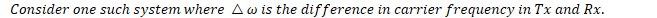The transmitted and received signals can be written as follows: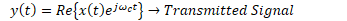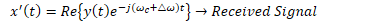As can be seen from above equation, the received signal's phase is time dependent.

So basically, CFO is causing a phase shift in time domain which reflects as frequency shift in Frequency Domain.

After sampling, the received signal will be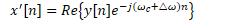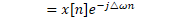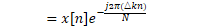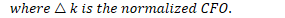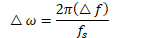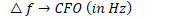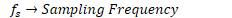Taking the FFT of this signal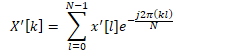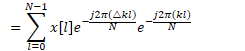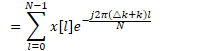The above equation can also be written as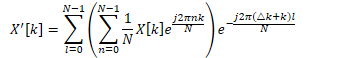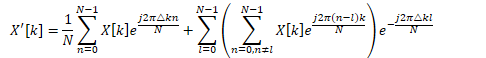The first term will be for n=l and the second term will occur when n≠l.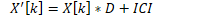D is the distortion (like scaling)

ICI is Inter carrier Interference

As seen from above analysis, CFO introduces Distortion and Inter Carrier Interference

To see the impact of the CFO in frequency domain and how to estimate CFO in 5G NR, watch the following video:

Impact of CFO can be seen in MATLAB

Effect of CFO on constellation

Normalized CFO (Δk) = 0.1Normalized CFO (Δk) = 0.2﻿